Question

A flat, rectangular coil consisting of 60 turns measures 23.0 cmcm by 34.0 cmcm . It is in a uniform, 1.40-TT, magnetic field, with the plane of the coil parallel to the field. In 0.230 ss , it is rotated so that the plane of the coil is perpendicular to the field.
(a) What is the change in the magnetic flux through the coil due to the rotation?
(b) What is the magnitude of the average emf induced in the coil during the rotation?
(c) What is the average current induced in the coil during the rotation?

1.(a)   ΔФ = -0.109W

(b)  emf = 28.43V

(c)   Iin = emf/R

Explanation:

(a) In order to calculate the magnetic flux you use the following formula: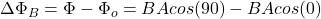(1)

B: magnitude of the magnetic field = 1.40T

A: area of the rectangular coil = (0.23m)(0.34m)=0.078m^2

Where it has been taken into account that at the beginning the normal vector to the cross sectional area of the coil, and the magnetic field vector are parallel. When the coil is rotated the vectors are perpendicular.

Then, you obtain: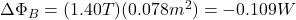The change in the magnetic flux is -0.109 W

(b) During the rotation of the coil the emf induced is given by: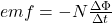(2)

N: turns of the coil = 60

ΔФ: change in the magnetic flux = 0.109W

Δt: lapse time of the rotation = 0.230s

You replace the values of the parameters in the equation (2):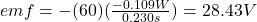The induced emf is 28.43V

(c) The induced current in the coil is given by: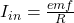(3)

R: resistance of the coil     (it is necessary to have this value)

emf :induced emf  = 28.43V